# Implementation of Frequency-Domain Simulation

The example code in Table 4.2 uses MathCad syntax, although it could easily be rewritten into any mathematical spreadsheet notation. The MathCad symbol := means the variable on the left is assigned the value of the expression on the right.

The example in Table 4.2 simulates a pulse of length N /2, with Gaussian rising and falling edges having 10% to 90% rise/fall time equal to 40 ps (4 D T ), and a delay equal to 4096 ps ((1/10) N D T ). These factors were implemented using definitions PulN , Gaus , and Dly from Table 4.1. Such a specification might well represent the differential output of a driver with 1-V amplitude and 40-ps rise/fall time (see Figure 4.4).

Figure 4.4. The time-domain signal x n shows a pulse of length (1/2) N D T , offset by a delay of 4096 ps = (1/10) N D T . The inset reveals a Gaussian rising edge with a 10% to 90% risetime of 40 ps (four samples).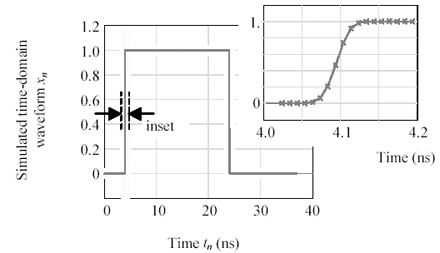Assuming the differential driver is connected in a system configuration as shown in Appendix C, "Two-Port Analysis," the system gain G may be computed, sampled on the dense grid of frequencies w k to produce a frequency-domain vector G k , and then multiplied point-by-point times the vector X k . The frequency-domain result, once inverse-transformed to the time domain, represents the response of system G to the stimulus X . The time-domain vector y will show the effects of all resistive losses, dielectric losses, bulk transport delay, and reflections within the transmission environment defined by G .

Equation 4.17Equation 4.18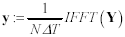Table 4.2. Example Code Showing FFT Simulation

Item

Expression

Units

Sampling resolution

D T := 10 “11

sec

Length of sample vector

N := 4096

a power of two

Index to time points

n := 0,1..( N “1)

integer

Horizontal axis for time-domain plots

t n := n D T

sec

Index to frequency points

k := 0,1..( N /2)

integer

Horizontal axis for frequency-domain plots

f k := k/ ( N D T )

Hertz

Frequencies used to sample Fourier transform functions

w k := 2 p f k , k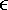0,1..( N /2)

Pulse of width ( N/ 2) D T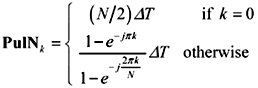vector

Delay operator (delays by amount t )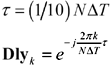vector

Gaussian LPF with 10% to 90% rise/fall time equal to 4 D T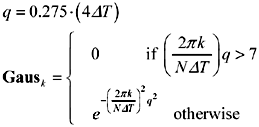vector

Example definition of signal in the frequency domain

X k := PulN k · Gaus k · Dly k

vector

Inverse transformation of frequency-domain vector X to produce time-domain vector x (see Figure 4.4)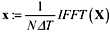vectorHigh-Speed Signal Propagation[c] Advanced Black Magic
ISBN: 013084408X
EAN: N/A
Year: 2005
Pages: 163